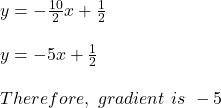Question

CAN SOMEONE HELP ME ASAP????

Find the gradient of the line 2y = -10x + 1

1.It A because the bike goes far away

2.Step-by-step explanation:

The gradient of a line is the slope of the line.

The standard form of the line equation is y = mx  + b

where m is the slope ( or gradient) b is the y – intercept.

Given :

2y = -10x + 1